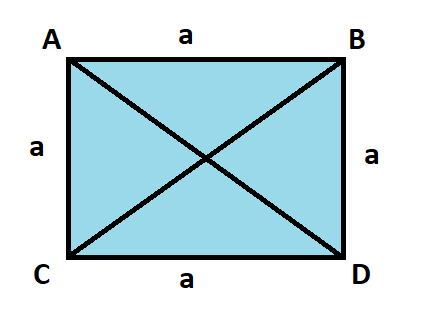# Diagonal Of A Square Formula

In geometry, a square is a regular quadrilateral that has four equal sides and four angles which are

$$\begin{array}{l}90^{\circ}\end{array}$$
in measurement. Thus the sum of all the internal angles of a square is equal to
$$\begin{array}{l}360^{\circ}\end{array}$$
.

Diagonal of a square-

A square can have two diagonals. Each of the diagonal can be formed by joining the diagonally opposite vertices of a square. The properties of diagonals are as follows-

• Both the diagonals are congruent (same length).
• Both the diagonals bisect each other, i.e. the point of joining of the two diagonals is the midpoint of both the diagonals.
• A diagonal divides a square into two isosceles right-angled triangles.

Length of the Diagonal-

Consider a square ABCD having sides equal to ‘a’ cm.

Let AD and BC be the two diagonals of a square.It is clearly visible that a diagonal divides the square into two right triangle, i,e,

$$\begin{array}{l}\bigtriangleup ACD\end{array}$$
and
$$\begin{array}{l}\bigtriangleup BDC\end{array}$$
.

Let us take any triangle of the two for calculating the length of the diagonal.

In

$$\begin{array}{l}\bigtriangleup ACD\end{array}$$
, applying the Pythagoras Theorem to obtain the diagonal length, we have

$$\begin{array}{l}AD^{2} = AC^{2} + CD^{2}\end{array}$$
……………(i)

we know AC = CD = a, …………………….(ii)

Substituting the value of (ii) in (i), we have

$$\begin{array}{l}AD^{2} = a^{2} + a^{2}\end{array}$$
$$\begin{array}{l}AD^{2} = 2a^{2}\end{array}$$
$$\begin{array}{l}\Rightarrow AD = \sqrt{2}a\end{array}$$

Thus the length of the diagonal of the given square is-

$$\begin{array}{l}AD = BC = \sqrt{2}a\end{array}$$

Example 1- Find the length of a diagonal of a square whose side is 4 cm.

Solution- Given a = 4 cm

Length of a diagonal =

$$\begin{array}{l}4 \sqrt{2} cm\end{array}$$

Example 2- Find the length of a diagonal of a square, given its area to be 64

$$\begin{array}{l}cm^{2}\end{array}$$
.

Solution- Given Area = 64

$$\begin{array}{l}cm^{2}\end{array}$$

We know, Area =

$$\begin{array}{l}a^{2}\end{array}$$
$$\begin{array}{l}a^{2} = 64\end{array}$$
$$\begin{array}{l}\Rightarrow a = 8\end{array}$$

Now Length of a diagonal =

$$\begin{array}{l}a\sqrt{2} = 8\sqrt{2} cm\end{array}$$